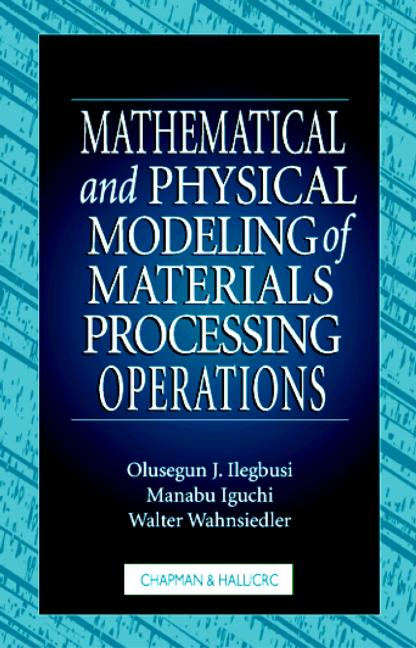# Mathematical and Physical Modeling of Materials Processing Operations

## 1st Edition

CRC Press

512 pages

##### Purchasing Options:\$ = USD
Hardback: 9781584880172
pub: 1999-07-29
SAVE ~\$48.00
Currently out of stock
\$240.00
\$192.00
x

FREE Standard Shipping!

### Description

The past few decades have brought significant advances in the computational methods and in the experimental techniques used to study transport phenomena in materials processing operations. However, the advances have been made independently and with competition between the two approaches. Mathematical models are easier and less costly to implement, but experiments are essential for verifying theoretical models.

In Mathematical and Physical Modeling of Materials Processing Operations, the authors bridge the gap between mathematical modelers and experimentalists. They combine mathematical and physical modeling principles for materials processing operations simulation and use numerous examples to compare theoretical and experimental results.

The modeling of transport processes is multi-disciplinary, involving concepts and principles not all of which can be associated with just one field of study. Therefore, the authors have taken care to ensure that the text is self-sustaining through the variety and breadth of topics covered. Beyond the usual topics associated with transport phenomena, the authors also include detailed discussion of numerical methods and implementation of process models, software and hardware selection and application, and representation of auxiliary relationships, including turbulence modeling, chemical kinetics, magnetohydrodynamics, and multi-phase flow. They also provide several correlations for representing the boundary conditions of fluid flow, heat transfer, and mass transfer phenomena.

Mathematical and Physical Modeling of Materials Processing Operations is ideal for introducing these tools to materials engineers and researchers. Although the book emphasizes materials, some of the topics will prove interesting and useful to researchers in other fields of chemical and mechanical engineering.

### Table of Contents

INTRODUCTION

Introductory Remarks

Mathematical Modeling

Physical Models

Pilot Plant

BUILDING BLOCKS OF MATHEMATICAL MODELS

Introduction

General Philosophy of Model Development

Some Basic Physical Situations of Relevance to Modeling

SCIENCE BASE OF MATHEMATICAL MODEL DEVELOPMENT

Introduction

Fluid Flow Phenomena

Heat Transfer

Diffusion and Mass Transfer

Multiphase Flow

SUBSIDIARY RELATIONSHIPS USED IN MATHEMATICAL MODELING

Introduction

Chemical Equilibria and Chemical Kinetics

Electromagnetic Flow Phenomena

Non-Newtonian Rheology of Semisolid Slurry Systems

Turbulence Modeling

Useful Correlations

Determination of Physical Properties

SCALING, SCOPING, AND ASYMPTOTIC SOLUTIONS

Introduction

Scaling

Scoping and Asymptotic Solutions

NUMERICAL TECHNIQUES

Introduction

Linear Equations

Nonlinear Equations

Functional Approximation

Numerical Differentiation

Numerical Integration

Ordinary Differential Equations

Partial Differential Equations

Finite Difference Method

Finite Element Method

Orthogonal Collocation

Boundary Integral Method

Optimization

IMPLEMENTATION AND VERIFICATION OF PROCESS MODELS

Introduction

Hardware

Software

Artificial Intelligence

The Future of Modeling Implementations

Verification of Models

MATHEMATICAL MODELING CASE STUDIES

Introduction

Scaling and Scoping-Electrolytic Deposition

Simulations of Fluid Flow and Heat Transfer in Coextrusion

Infiltration Processing of Silicon Carbide

Stoichiometry Driven Marangoni Convection in GaAs Growth

Czochralski Crystal Growth

Flow Characteristics in Gas-Agitated Bath

BASIC ELEMENTS OF PHYSICAL MODELING

Principles of Physical Modeling

Flow Visualization Technique

Measurement of Turbulent Phenomena

Bubble Characteristics

Measurement of Interfacial Phenomena

Measurement of Heat and Mass Transfer

PHYSICAL MODELING EXAMPLES

Bubbling and Jetting

Mixing

Swirl Motion of Bubbling jet

Bubble Characteristics

Liquid Flow Characteristics in a Bath Agitated by Gas Injection

Heat Transfer Between Bubbles and Molten Metal

Heat Transfer Between Solid Body and Molten Metal

Mass Transfer Between Bubbles and Liquid

Mass Transfer Between a Solid Body and Liquid

Entrainment of Top Slag by Lower Molten Metal

Effects of Top Slag on Liquid Flow Characteristics

Turbulence Structure in Bottom Blown Bath with Top Slag Using Conditional Sampling

APPENDICES

Dimensionless Numbers Useful in Process Metallurgy

Fluid Flow Equations in Various Coordinate Systems

Components of the Stress Tensor for Newtonian Fluids in Various Coordinate Systems

The Viscous Dissipation Term for Newtonian Fluids in Various Coordinate Systems

Plus each chapter includes references

### Subject Categories

##### BISAC Subject Codes/Headings:
MAT003000
MATHEMATICS / Applied
TEC009070
TECHNOLOGY & ENGINEERING / Mechanical
TEC021000
TECHNOLOGY & ENGINEERING / Material Science